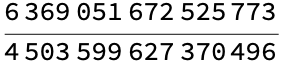# Wolfram CloudResource Object

Function Resource

# RealRationalize

Express a real number as a nearby rational number

 ResourceFunction["RealRationalize"][r] expresses real number r as a rational number p/q.

## Details and Options

ResourceFunction["RealRationalize"] uses RealDigits to compute its answer.
Unlike Rationalize which returns rational numbers only under certain conditions, ResourceFunction["RealRationalize"] returns a rational numbers for most real number inputs.
ResourceFunction["RealRationalize"] works with complex numbers by operating on its real and imaginary parts directly.

## Examples

### Basic Examples (3)

Express 0.5 as a rational number:

 In:=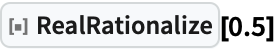Out=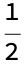Express the machine precision approximation of π as a rational number:

 In:=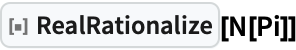Out=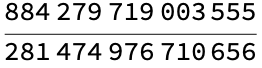Express a 50-digit approximation of π as a rational number:

 In:=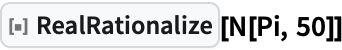Out=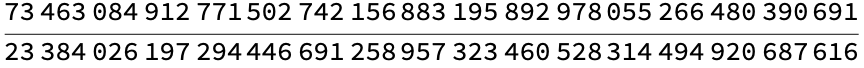### Scope (5)

Integers are returned unchanged:

 In:=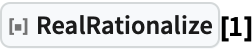Out=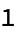Rationals are returned unchanged:

 In:=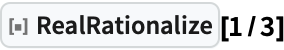Out=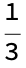Exact representations of irrational numbers are returned unchanged:

 In:=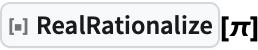Out=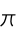RealRationalize also works with complex numbers by applying itself to the real and imaginary parts of that number:

 In:=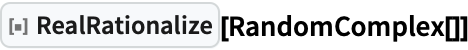Out=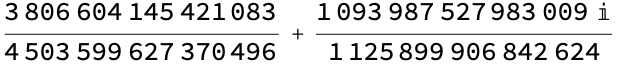RealRationalize has the Listable attribute, so it automatically works with lists:

 In:=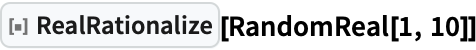Out=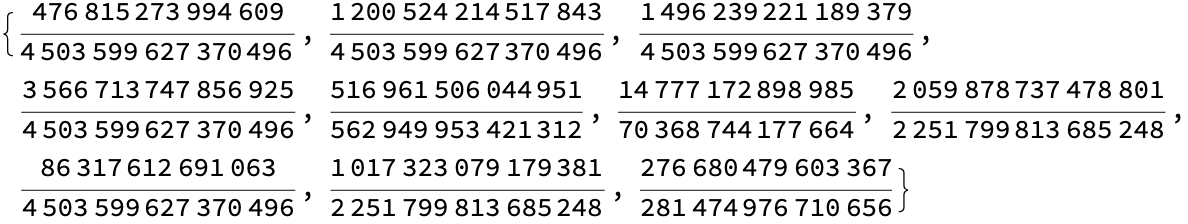### Properties and Relations (4)

Converting the rational representation back to a real number typically yields the original real number:

 In:=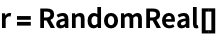Out=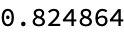In:=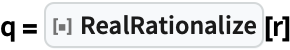Out=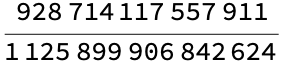In:=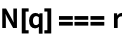Out=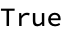Unlike Rationalize, which has specific constraints on which numbers are rationalized and which ones are not, RealRationalize always attempts to return the nearest a rational number using RealDigits as its guide:

 In:=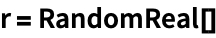Out=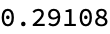In:=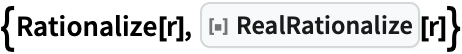Out=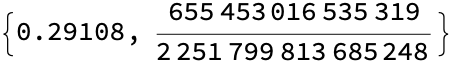RealRationalize simply finds a close approximation, whereas Rationalize aims to find a human-friendly approximation:

 In:=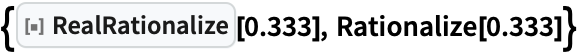Out=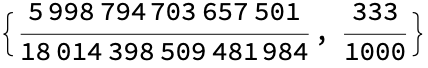In:=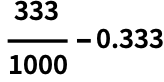Out=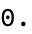In:=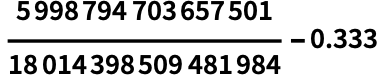Out=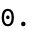RealRationalize may give slightly "better" results than Rationalize for certain special real number inputs:

 In:=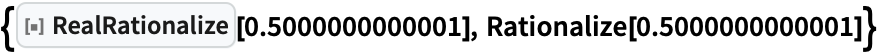Out=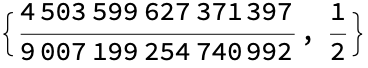In:=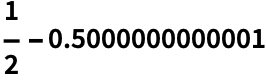Out=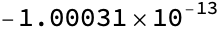In:=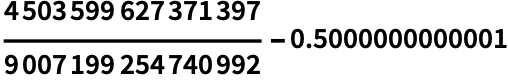Out=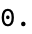### Possible Issues (2)

No attempt is made to rationalize exact representations of irrational numbers:

 In:=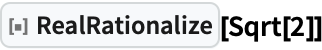Out=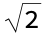Convert these numbers to a machine or high-precision real first:

 In:=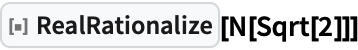Out=